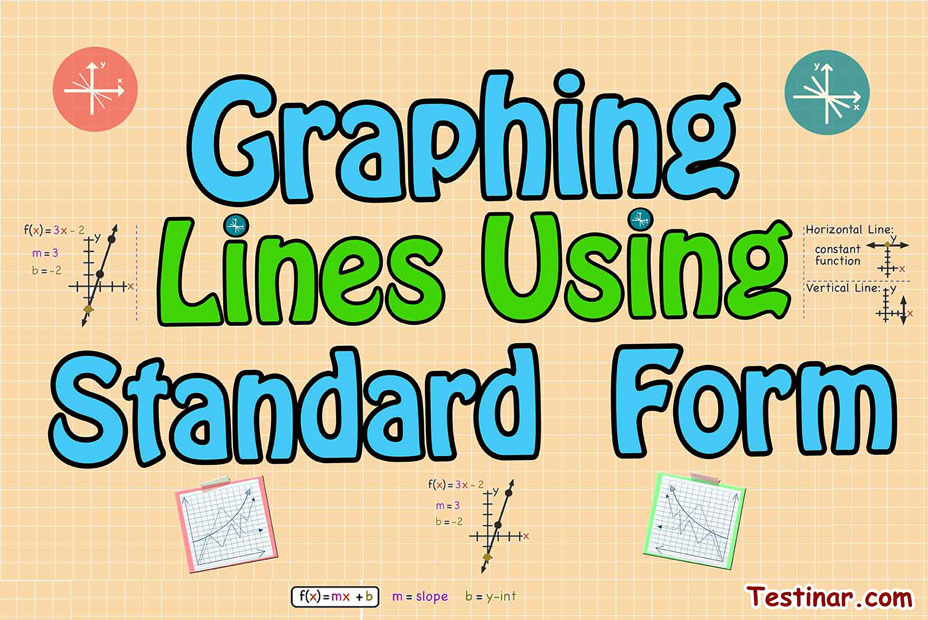## How to Graph Lines Using Standard Form?

There are lots of ways to express the equation of a line. There's slope-intercept form, point-slope form, and additionally the topic of this page. Each of them expresses the equation of a line, as well as each of them, has its own advantages and disadvantages. For example, the point-slope form makes it simple for finding the line's equation whenever one just knows the slope as well as a single point on the line. Standard form additionally has a few distinct uses, however, we will cover more on it later.

## Define Standard Form?

Standard Form is shown as: $$Ax \ + \ By \ = \ C \ , \ A \ ≠ \ 0 \ , \ B \ ≠ \ 0$$
Where $$A$$ and $$B$$ are coefficients and $$C$$ is a constant.

Examples:

• $$2x \ + \ 4y \ = \ 8$$
• $$5x \ - \ 7y \ = \ 12$$
• $$3x \ - \ 9y \ = \ -18$$

If the equation is given in standard form, one can’t identify the slope and $$y$$-intercept needed for graphing.

Therefore... what is done?

There are really $$2$$ different methods that can be used to graph linear equations written in standard form. One can utilize either one, so here’s both of them and you can decide which one you like best.

Here is an example solved by $$2$$ methods.

### Method one: Rewriting an Equation in Slope Intercept Form

For this lesson, we will find out the way to convert a standard form equation into an equation written in slope-intercept form.
So, let’s begin! If you’ve got a standard form equation, it can be rewritten in slope-intercept form.

The objective whenever you rewrite a standard form equation in slope intercept form is to redo the equation so it reads as $$y \ = \ mx \ + \ b$$. You’ll have to utilize your knowledge of solving equations. Don’t forget to utilize opposite operations and whatever is done to one side of an equation, must be done to the other side.
So we can redo $$6x \ - \ 2y \ = \ 4$$ in slope-intercept form so we can graph the equation easily.

Step1: Isolate $$y$$ for $$x$$ by Subtracting $$6x$$ from both sides.
$$6x \ - \ 2y \ \color{red}{-6x} \ = \ 4 \ \color{red}{-6x}$$

Step2: Rewrite the right side of the equation.
$$-2y \ = \ 4 \ \color{red}{-6x}$$

Step3: Divide all the terms by the coefficient of $$y$$.
$$\frac{-2y}{\color{red}{-2}} \ = \ \frac{4}{\color{red}{-2}} \ - \ \frac{6x}{\color{red}{-2}}$$

Step4: If possible, simplify.
$$y \ = \ 3x \ - \ 2$$

Step5: Graph the line.
$$y$$-intercept $$= \ -2$$ , $$Slop \ = \ 3$$: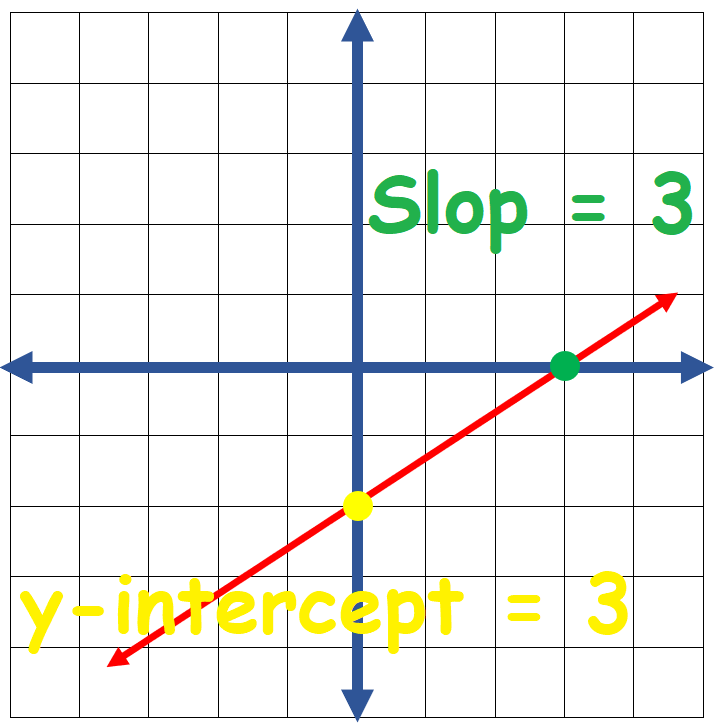See the way making a graph is fairly simple after it gets written in a slope-intercept form. It’s always possible to redo standard form equations in a slope-intercept form. Be certain you solve the equation for $$y$$, and then you are done!

### Method two: Utilizing X and Y Intercept for Graphing Linear Equations

Now you know one method of graphing standard form equations – via converting it into a slope-intercept form. But there’s also an additional method of graphing standard form equations, which is to locate its $$x$$ and $$y$$ intercepts. So, let’s go over what the word intercepts means. Intercept is where your line crosses an axis. You have an $$x$$, as well as a $$y$$ intercept.

The point where a line touches the $$x$$-axis is known as an $$x$$-intercept. The point where the line touches the $$y$$-axis is known as a $$y$$-intercept. If you can locate the points where a line crosses an $$x$$ and $$y$$-axis, after that you get 2 points and can draw a line.
Whenever equations are presented in standard form, it’s fairly simple to locate intercepts.

For graphing a linear equation utilizing $$X$$ and $$Y$$ Intercept you must do the following steps:

• Locate the $$x$$-intercept of the line via using zero for $$y$$.
• Locate the $$y$$- intercept of the line via using a zero for the $$x$$.
• Connect the $$2$$ points.

Now let's see another Example

Draw a graph of $$6x \ - \ 3y \ = \ -6$$.

• Firstly isolate $$y$$ for $$x$$: $$6x \ - \ 3y \ = \ -6 \ → \ -3y \ = \ -6x \ -6 \ → \ y \ = \ 2x \ + \ 2$$
• Locate the $$x$$−intercept of the line via using a zero for $$y$$. $$2x \ + \ 2 \ = \ y \ → \ 2x \ + \ 2 \ = \ 0 \ → \ 𝑥 \ = \ \frac{-2}{2} \ = -1$$
• Locate the $$y$$−intercept of the line via using a zero for the $$x$$. $$2x \ + \ 2 \ = \ y \ → \ 2(0) \ + \ 2 \ = \ y \ → \ y \ = \ 2$$
• After that: $$x$$−intercept: $$(−1,0)$$ and $$y$$−intercept: $$(0,2)$$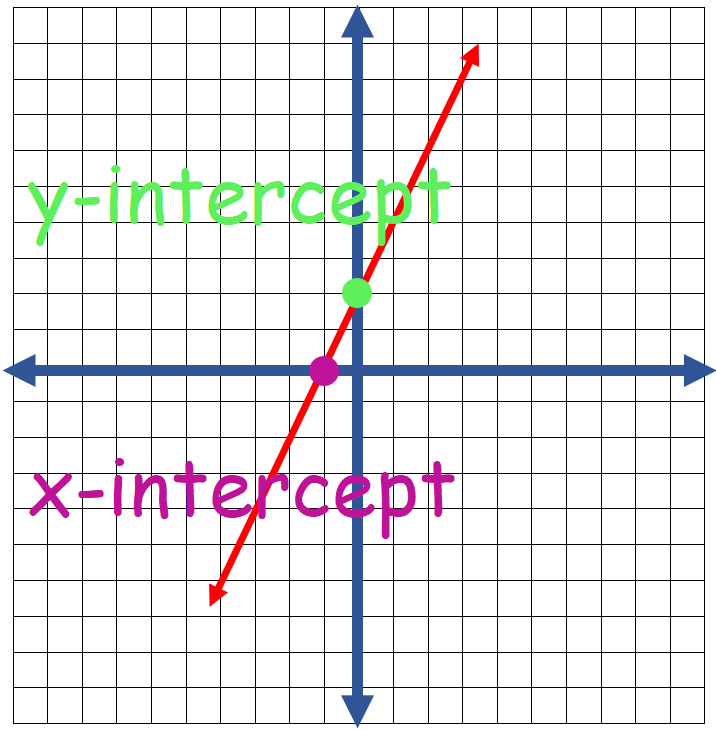### Exercises for Graphing Lines Using Standard Form

1) Sketch the graph of the line: $$3y \ - \ 6x \ = \ 3$$

2) Sketch the graph of the line: $$\frac{1}{3} \ y \ - \ x \ = \ -\frac{2}{3}$$

3) Sketch the graph of the line: $$-6y \ + \ 6x \ = \ 30$$

4) Sketch the graph of the line: $$-5y \ + \ \frac{5}{2} \ x \ = \ -15$$

5) Sketch the graph of the line: $$11y \ + \ 22x \ = \ 11$$

6) Sketch the graph of the line: $$2y \ + \ 3x \ = \ -4$$

7) Sketch the graph of the line: $$-7y \ - \ 7x \ = \ 14$$

8) Sketch the graph of the line: $$3y \ + \ 9x \ = \ 9$$

9) Sketch the graph of the line: $$-6y \ - \ 4x \ = \ -12$$

10) Sketch the graph of the line: $$-15y \ - \ 6x \ = \ 30$$

1) Sketch the graph of the line: $$3y \ - \ 6x \ = \ 3$$

$$\color{red}{3y \ - \ 6x \ = \ 3 \ ⇒ \ y \ = \ 2x \ + \ 1 \ ⇒}$$

 $$\color{red}{y}$$ $$\color{red}{x}$$ $$\color{red}{0}$$ $$\color{red}{-\frac{1}{2}}$$ $$\color{red}{1}$$ $$\color{red}{0}$$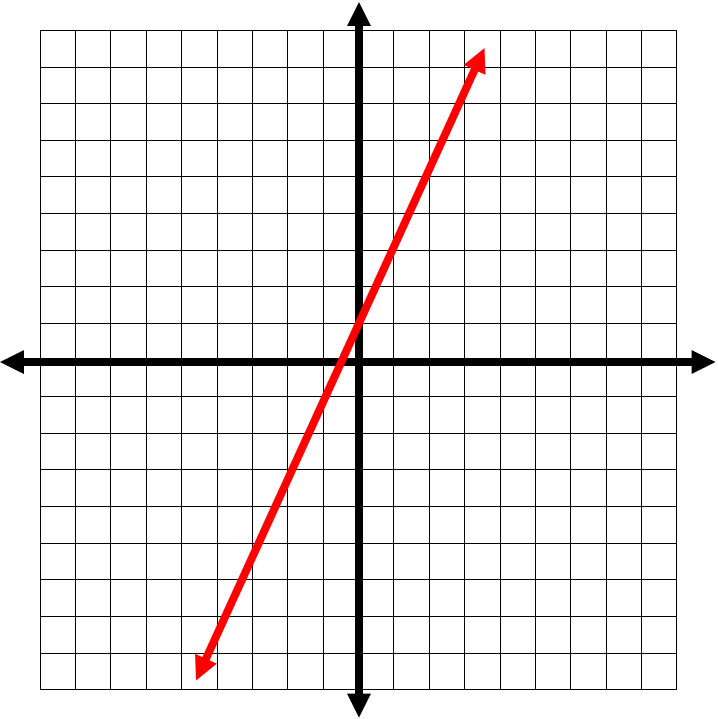2) Sketch the graph of the line: $$\frac{1}{3} \ y \ - \ x \ = \ -\frac{2}{3}$$

$$\color{red}{\frac{1}{3} \ y \ - \ x \ = \ -\frac{2}{3} \ ⇒ \ y \ = \ 3x \ - \ 2 \ ⇒}$$

 $$\color{red}{y}$$ $$\color{red}{x}$$ $$\color{red}{0}$$ $$\color{red}{\frac{2}{3}}$$ $$\color{red}{-2}$$ $$\color{red}{0}$$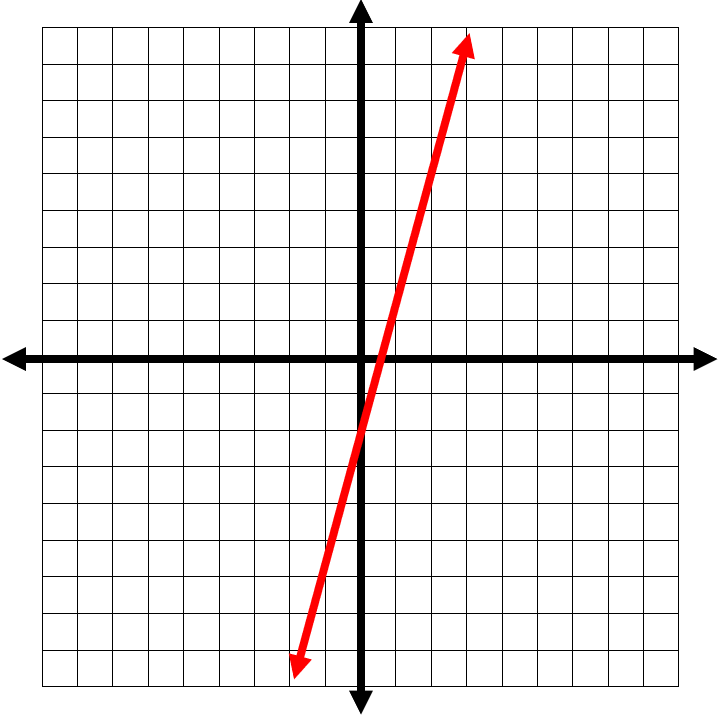3) Sketch the graph of the line: $$-6y \ + \ 6x \ = \ 30$$

$$\color{red}{-6y \ + \ 6x \ = \ 30 \ ⇒ \ y \ = \ x \ - \ 5 \ ⇒}$$

 $$\color{red}{y}$$ $$\color{red}{x}$$ $$\color{red}{0}$$ $$\color{red}{5}$$ $$\color{red}{-5}$$ $$\color{red}{0}$$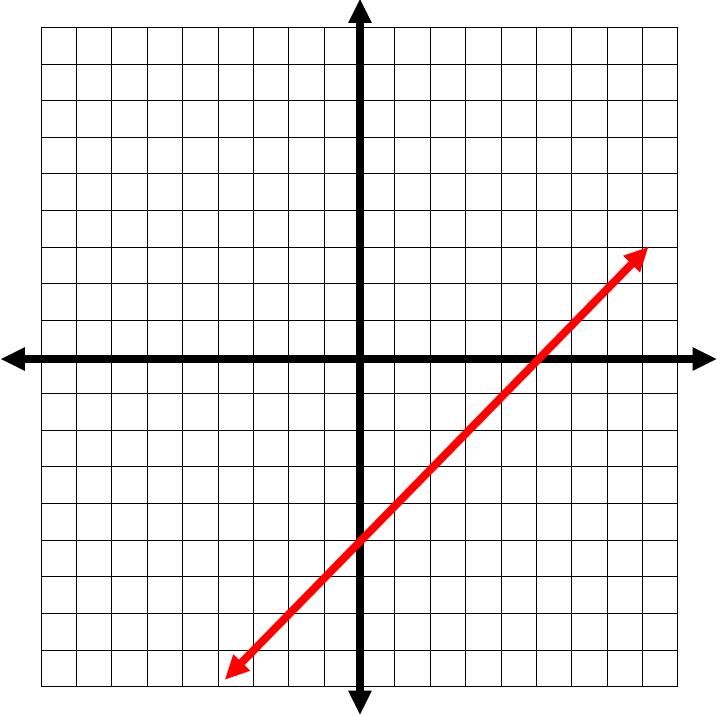4) Sketch the graph of the line: $$-5y \ + \ \frac{5}{2} \ x \ = \ -15$$

$$\color{red}{-5y \ + \ \frac{5}{2} \ x \ = \ -15 \ ⇒ \ y \ = \ \frac{1}{2} \ x \ + \ 3 \ ⇒}$$

 $$\color{red}{y}$$ $$\color{red}{x}$$ $$\color{red}{0}$$ $$\color{red}{-6}$$ $$\color{red}{3}$$ $$\color{red}{0}$$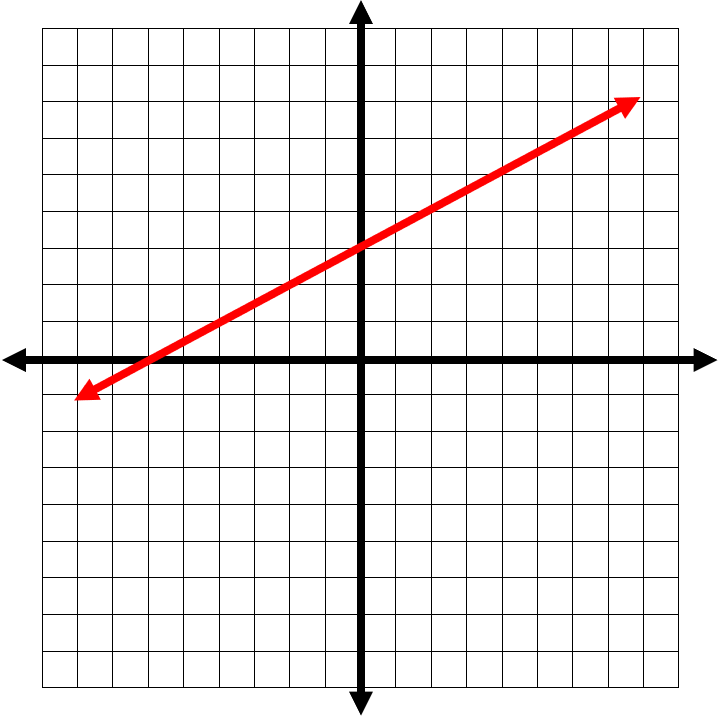5) Sketch the graph of the line: $$11y \ + \ 22x \ = \ 11$$

$$\color{red}{11y \ + \ 22x \ = \ 11 \ ⇒ \ y \ = \ -2x \ + \ 1 \ ⇒}$$

 $$\color{red}{y}$$ $$\color{red}{x}$$ $$\color{red}{0}$$ $$\color{red}{\frac{1}{2}}$$ $$\color{red}{1}$$ $$\color{red}{0}$$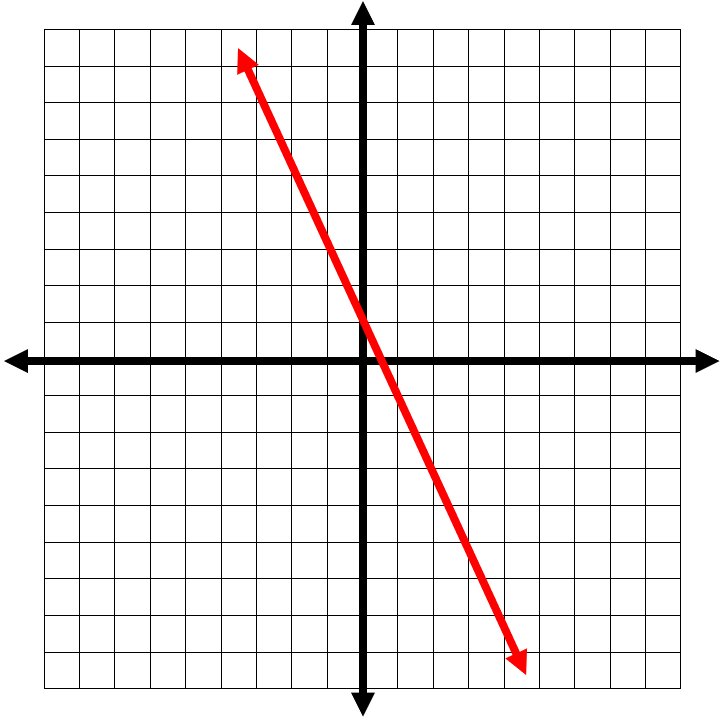6) Sketch the graph of the line: $$2y \ + \ 3x \ = \ -4$$

$$\color{red}{2y \ + \ 3x \ = \ -4 \ ⇒ \ y \ = \ -\frac{3}{2} \ x \ - \ 2 \ ⇒}$$

 $$\color{red}{y}$$ $$\color{red}{x}$$ $$\color{red}{0}$$ $$\color{red}{-1 \ \frac{1}{3}}$$ $$\color{red}{-2}$$ $$\color{red}{0}$$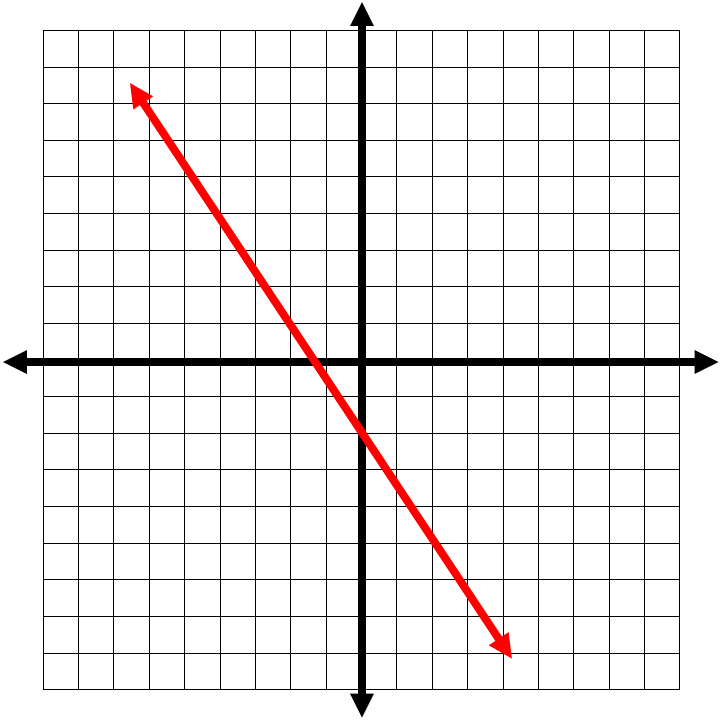7) Sketch the graph of the line: $$-7y \ - \ 7x \ = \ 14$$

$$\color{red}{-7y \ - \ 7x \ = \ 14 \ ⇒ \ y \ = \ -x \ - \ 2 \ ⇒}$$

 $$\color{red}{y}$$ $$\color{red}{x}$$ $$\color{red}{0}$$ $$\color{red}{-2}$$ $$\color{red}{-2}$$ $$\color{red}{0}$$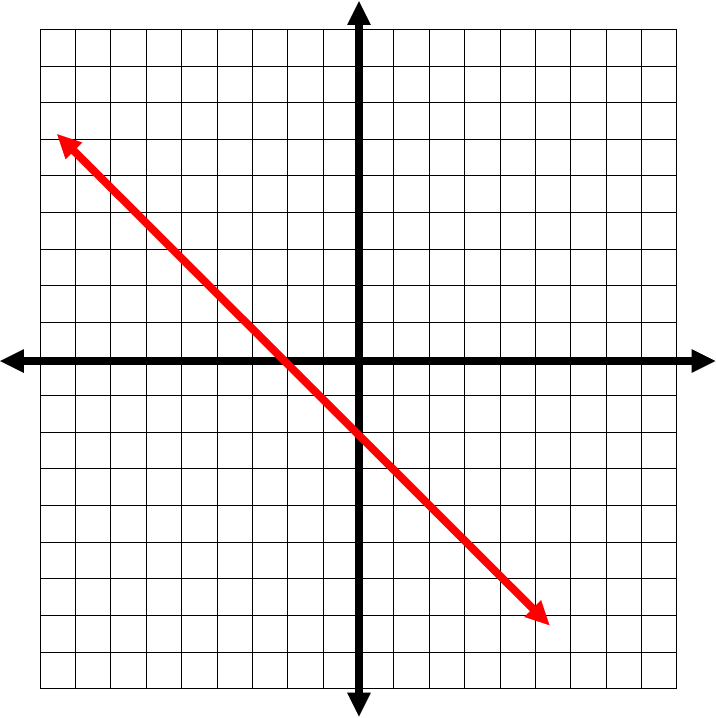8) Sketch the graph of the line: $$3y \ + \ 9x \ = \ 9$$

$$\color{red}{3y \ + \ 9x \ = \ 9 \ ⇒ \ y \ = \ -3x \ + \ 3 \ ⇒}$$

 $$\color{red}{y}$$ $$\color{red}{x}$$ $$\color{red}{0}$$ $$\color{red}{1}$$ $$\color{red}{3}$$ $$\color{red}{0}$$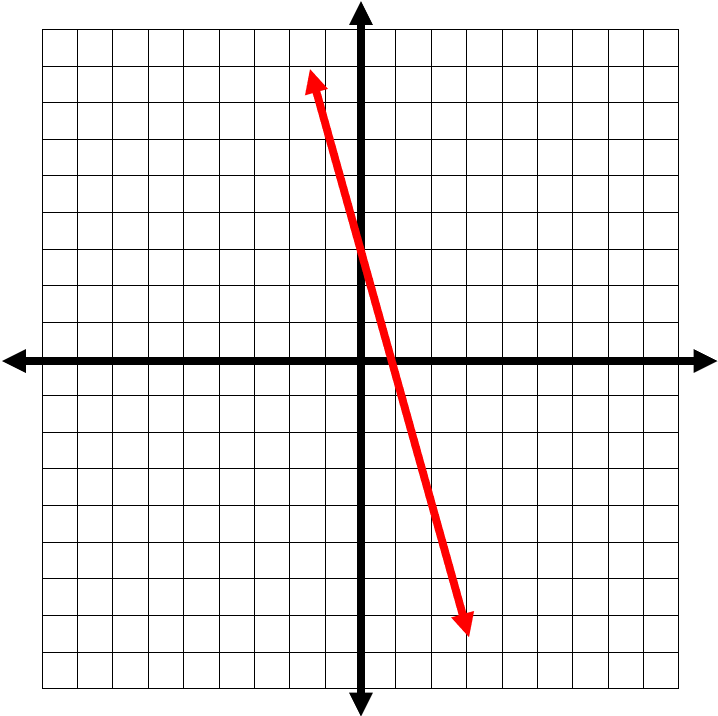9) Sketch the graph of the line: $$-6y \ - \ 4x \ = \ -12$$

$$\color{red}{-6y \ - \ 4x \ = \ -12 \ ⇒ \ y \ = \ -\frac{2}{3} \ x \ + \ 2 \ ⇒}$$

 $$\color{red}{y}$$ $$\color{red}{x}$$ $$\color{red}{0}$$ $$\color{red}{3}$$ $$\color{red}{2}$$ $$\color{red}{0}$$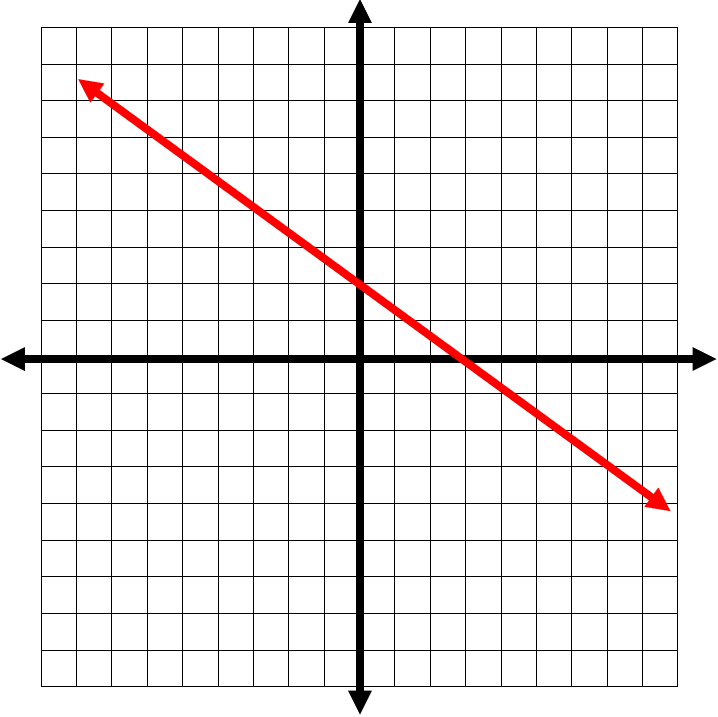10) Sketch the graph of the line: $$-15y \ - \ 6x \ = \ 30$$

$$\color{red}{-15y \ - \ 6x \ = \ 30 \ ⇒ \ y \ = \ -\frac{2}{5} \ x \ - \ 2 \ ⇒}$$

 $$\color{red}{y}$$ $$\color{red}{x}$$ $$\color{red}{0}$$ $$\color{red}{-5}$$ $$\color{red}{-2}$$ $$\color{red}{0}$$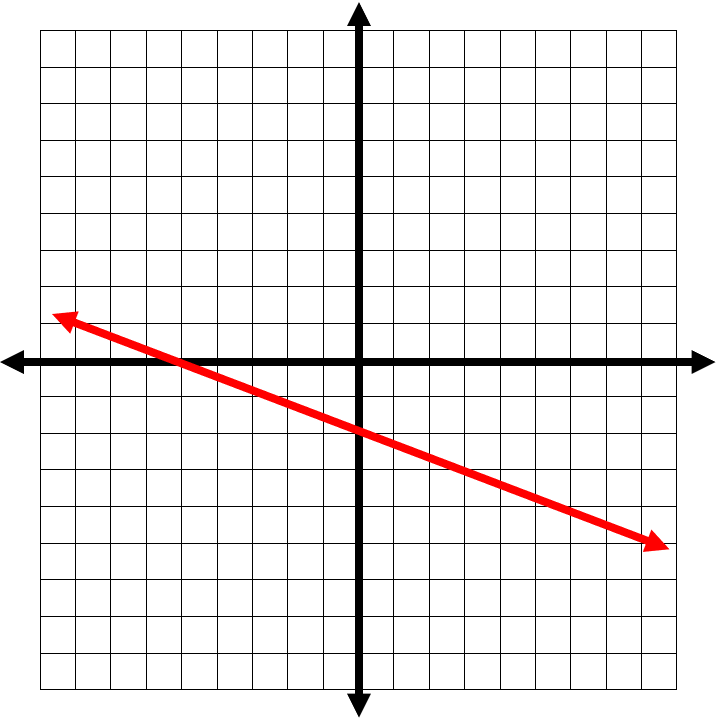## Graphing Lines Using Standard Form Quiz

### ACT Math for Beginners 2022

$24.99$13.99

### Comprehensive CLEP College Algebra Practice

$19.99$14.99

### GED Math Full Study Guide 2022-2023

$25.99$13.99

### Accuplacer Math Full Study Guide

$25.99$12.99